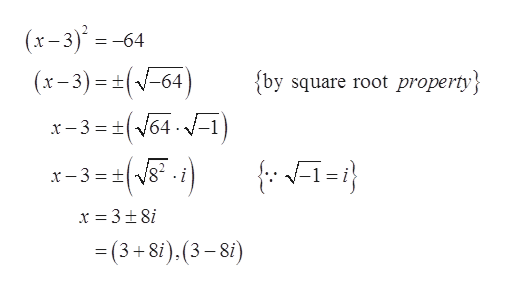Square root property

Question

Square root property

Step 1

To determine the roots of given equation.

Step 2

Given information: The equation is shown below.

Step 3

Apply square root...help_outlineImage Transcriptionclose(x-3)=-6- -64 ) (x-3)=(V-64 {by square root property x-3 64 =(3+8i).(3-8i) fullscreen

Want to see the full answer?

See Solution

Want to see this answer and more?

Our solutions are written by experts, many with advanced degrees, and available 24/7

See Solution
Tagged in

Algebra